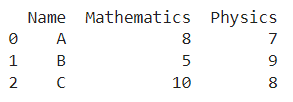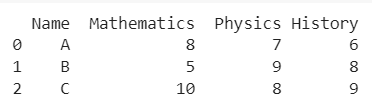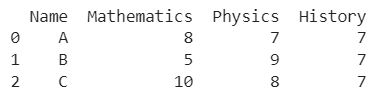# How To Add A New Column To An Existing DataFrame?

A DataFrame is the primary data structure of the Pandas library and is commonly used for storing and working with tabular data. A common operation that could be performed on such data is adding a new column to an existing dataframe in order to add more information to the data.

To start working with Pandas, we first need to import it to the Python code:

### Python 3 Code :

``import pandas as pd``

## Running Example

Let us understand this operation with the help of an example. Consider the following dataframe containing 3 students with names A, B, and C and their corresponding marks (out of 10) for two subjects, Mathematics and Physics.Code snippet for generating the above DataFrame :

### Python 3 Code :

``````import pandas as pd

# Dictionary for our data
data = {'Name' : ['A', 'B', 'C'], 'Mathematics' : [8, 5, 10], 'Physics' : [7, 9, 8]}

# DataFrame for the dictionary
df = pd.DataFrame(data)

# Printing the DataFrame
print(df)``````

Here, data is a dictionary we created to initialize the DataFrame. For this, we use the DataFrame() function of the Pandas library which takes the dictionary as an argument and returns the required DataFrame.

Now, let’s say we need to add the marks of another subject, ‘History’, to this dataframe as shown below.Let us look at different ways to do this :

## Add A New Column To An Existing DataFrame Using a list

This method is pretty straightforward and is the most commonly used one. The syntax can be seen below, with ‘History’ being the new column’s label and [6,8,9] being a list denoting row-wise values for that column.

The resulting new column is added as the last one in the dataframe. This method is used for ‘in-place’ addition of a column in the DataFrame.

Python 3 Code :

``````import pandas as pd

# Dictionary for our data
data = {'Name' : ['A', 'B', 'C'], 'Mathematics' : [8, 5, 10], 'Physics' : [7, 9, 8]}

# DataFrame for the dictionary
df = pd.DataFrame(data)

# Adding a new column named History
df['History'] = [6, 8, 9]

# Printing the new dataframe
print(df)``````

Output :## Add A New Column To An Existing DataFrame Using the insert() function

In this method, we use the insert() function to add a new column to an existing dataframe. This function can be used to add the column at any position, not necessarily at the end of the dataframe.

This method is used for ‘in-place’ addition of a column in the DataFrame.The new column’s index, label, and data can be specified as function arguments as follows (3, ‘History’ and [6,8,9] respectively here) :

Python 3 Code :

``````import pandas as pd

# Dictionary for our data
data = {'Name' : ['A', 'B', 'C'], 'Mathematics' : [8, 5, 10], 'Physics' : [7, 9, 8]}

# DataFrame for the dictionary
df = pd.DataFrame(data)

# Adding a new column named History
df.insert(3, 'History', [6,8,9])

# Printing the new dataframe
print(df)``````

Output :Instead of having [6,8,9] as values for the rows for that particular column, if we want all the rows to have the same value (say 7), we can specify it in the arguments as follows :

Python 3 Code :

``````import pandas as pd

# Dictionary for our data
data = {'Name' : ['A', 'B', 'C'], 'Mathematics' : [8, 5, 10], 'Physics' : [7, 9, 8]}

# DataFrame for the dictionary
df = pd.DataFrame(data)

# Adding a new column named History
df.insert(3, 'History', 7)

# Printing the new dataframe
print(df)``````

Output :If the label of the column to be added matches that of another column already present in the DataFrame, we receive an error. For such additions, it is better to use the assign() function instead.

## Add A New Column To An Existing DataFrame Using the assign() function

In this method, we use the assign() function to add a new column to an existing dataframe. This function returns the updated DataFrame. The syntax of the argument is as follows :

``<name_of_col_to_be_added> = <value>``

Note: Existing columns that are reassigned will be overwritten.

Python 3 Code :

``````import pandas as pd

# Dictionary for our data
data = {'Name' : ['A', 'B', 'C'], 'Mathematics' : [8, 5, 10], 'Physics' : [7, 9, 8]}

# DataFrame for the dictionary
df = pd.DataFrame(data)

# Adding a new column named History
df = df.assign(History = [6,8,9])

# Printing the new dataframe
print(df)``````

Output :Just like the previous method, if we want all the values in the new column to be the same (say 7), instead of a list, we can specify it in the arguments as follows :

Python 3 Code :

``````import pandas as pd

# Dictionary for our data
data = {'Name' : ['A', 'B', 'C'], 'Mathematics' : [8, 5, 10], 'Physics' : [7, 9, 8]}

# DataFrame for the dictionary
df = pd.DataFrame(data)

# Adding a new column named History
df = df.assign(History = 7)

# Printing the new dataframe
print(df)``````

Output :## Add A New Column To An Existing DataFrame Using the .loc property

In this method, we shall use the ‘.loc’ property of dataframes to add a new column. Through :(colon) we specify that we need to add values for all rows and in the second input we specify the required column label. ‘History’ here. This method is used for in-place addition of a column.

Python 3 Code :

``````import pandas as pd

# Dictionary for our data
data = {'Name' : ['A', 'B', 'C'], 'Mathematics' : [8, 5, 10], 'Physics' : [7, 9, 8]}

# DataFrame for the dictionary
df = pd.DataFrame(data)

# Adding a new column named History
df.loc[:, 'History'] = [6,8,9]

# Printing the new dataframe
print(df)``````

Output :## Add A New Column To An Existing DataFrame Using the eval() function

In this method, we use the eval() function to add a new column to the dataframe. We specify the argument as a string expression in the following manner :

``<new_col_label> = <new_col_value>``

The values of the new column can be specified using a list. [6,8,9] was used as an example for this purpose. The eval() function returns the updated DataFrame.

Python 3 Code :

``````import pandas as pd

# Dictionary for our data
data = {'Name' : ['A', 'B', 'C'], 'Mathematics' : [8, 5, 10], 'Physics' : [7, 9, 8]}

# DataFrame for the dictionary
df = pd.DataFrame(data)

# Adding a new column named History
df = df.eval('History = [6,8,9]')

# Printing the new dataframe
print(df)``````

Output :By default, the addition of a new column is not inplace. For inplace addition you must set the parameter inplace = True as follows :

Python 3 Code :

``````import pandas as pd

# Dictionary for our data
data = {'Name' : ['A', 'B', 'C'], 'Mathematics' : [8, 5, 10], 'Physics' : [7, 9, 8]}

# DataFrame for the dictionary
df = pd.DataFrame(data)

# Adding a new column named History
df.eval('History = [6,8,9]', inplace = True)

# Printing the new dataframe
print(df)``````

Output :## Conclusion

In this topic, we have learned to add a column to an existing Pandas DataFrame, following a running example of test scores of students in different subjects, thus giving us an intuition of how this concept could be applied in real-world situations. Feel free to reach out to [email protected] in case of any suggestions.

Trending